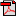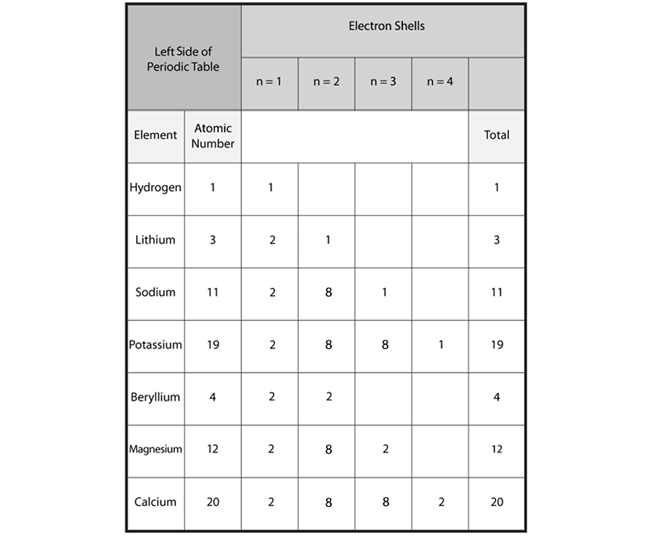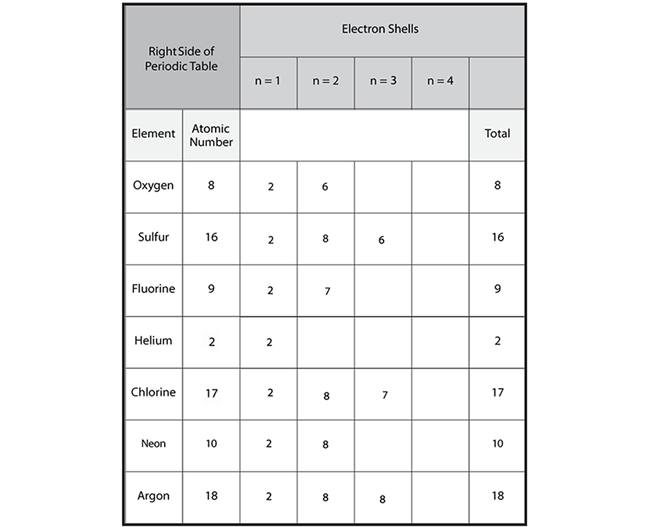# Lesson 6: The Recurring (Periodic) Table of Elements

## Activities

### Activity 1: Organization of the Periodic Table

Materials: periodic table of elements (kit)
In this first activity, you will be introduced to some basic information about the elements found on the periodic table. You will be looking at atomic number, atomic mass, and electron configuration (specifically the number of electrons on the outer shell). You will then complete a couple of activities that will help reinforce your understanding. NOTE: A full-color copy of the periodic table has been included with this unit. You should have it handy to refer to as needed.

Consider the "Carbon Example" graphic. The atomic number, which represents the number of protons in the element, is at the top of the information square. The name of the element is above the atomic number. The atomic mass of the element is at the bottom. In between each number is the shorthand abbreviation or symbol for each element.
Carbon ExampleKeep in mind the following information about the parts of the atom:
• A proton is a positively charged particle in the nucleus of the atom. The number of protons in an atom's nucleus is its atomic number.
• An electron is a negatively charged particle surrounding the nucleus of the atom.
• The number of electrons surrounding the nucleus of an atom is equal to the number of protons in the atom.
• A neutron is a particle in the nucleus that has about the same mass as a proton but has no charge. For the atoms of the first 20 elements, the number of neutrons is either equal to or slightly greater than the number of protons.
Using the information provided, complete the "Organization of the Periodic Table" page by filling in the missing information for each element in the table. After you complete the table, use the information to fill in the five blocks at the bottom of the page. Refer to the "Carbon Example" graphic as needed.Student Activity Page
Your child should fill out the chart provided with the following answers:### Activity 2: Periodic Table and Atomic Structure

In this activity, think back to the beginning of the unit when you worked on atomic structure and learned about the number of electrons on the outer shells of each element.

On the "Periodic Table and Atomic Structure" page, you will write the number of electrons present for each shell that is used. For example, helium has two electrons. They fill the first shell, so you will write "2" in the "n=1" column. If helium had three electrons, you would write 2 for the first shell and 1 for the second shell.

As you recall, each atom has orbitals (also called shells). In the list below and on the activity page, "n" represents which orbital is represented. The label n=1 represents the orbital closest to the nucleus; n=2 equals the orbital that is second closest, etc. Each orbital can hold a maximum number of electrons:
• 1st orbital (n =1) -- maximum number of electrons is 2
• 2nd orbital (n=2) -- maximum number of electrons is 8
• 3rd orbital (n=3) -- maximum number of electrons is 18*
• 4th orbital (n=4) -- maximum number of electrons is 32 (although the maximum number we will work with in this unit is 8)
*Note that although the 3rd orbital can hold a maximum of 18 electrons, the 4th orbital begins to fill before the 3rd orbital is full. For the elements we'll look at in this unit, the 3rd orbital will hold up to 8 electrons. After the 3rd orbital has 8 electrons, it is considered full, and the 4th orbital will begin filling. In high school chemistry, you will learn that the rules for filling shells 3 and 4 are more complicated for some elements.

Use the information about each orbital to complete the activity page. Remember that the number of electrons is equal to the number of protons. Once one orbital is full (has its maximum number of electrons), start filling the next orbital (the exception being the third shell — once it contains 8 electrons, you start filling the 4th orbital).

Hold on to your activity sheets. You will use them in other parts of the lesson, and you will use information from the table for your final activity.Student Activity Page
Periodic Table and Atomic Structure - Left Side of Periodic Table - KeyPeriodic Table and Atomic Structure - Right Side of Periodic Table - Key